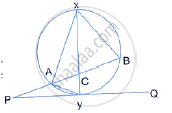Share

In the Given Figure, Xy is the Diameter of the Circle and Pq is a Tangent to the Circle at Y. If ∠Axb = 50° and ∠Abx = 70° and ∠Bay and ∠Apy - ICSE Class 10 - Mathematics

ConceptTangent Properties - If a Line Touches a Circle and from the Point of Contact, a Chord is Drawn, the Angles Between the Tangent and the Chord Are Respectively Equal to the Angles in the Corresponding Alternate Segments

Question

In the given figure, XY is the diameter of the circle and PQ is a tangent to the circle at Y.If ∠AXB = 50° and ∠ABX = 70° and ∠BAY and ∠APY

Solution

In ΔAXB,
∠XAB + ∠AXB + ∠ABX=180° [Triangle property]
⇒  ∠XAB + 50° + 70° = 180°
⇒  ∠XAB = 180° − 120° = 60°
⇒  ∠XAY=90° [Angle of semi-circle]
∴ ∠BAY = ∠XAY − ∠XAB = 90° − 60° = 30°
and  ∠BXY =  ∠BAY = 30° [Angle of same segment]
∠ACX =  ∠ BXY +  ∠ABX [External angle = Sum of two interior angles]
= 30° + 70°
= 100°
also,
∠XYP = 90° [Diameter ⊥ tangent]
∠ APY = ∠ACX − ∠CYP
∠APY = 100° − 90°
∠APY = 10°

Is there an error in this question or solution?

Video TutorialsVIEW ALL 

Solution In the Given Figure, Xy is the Diameter of the Circle and Pq is a Tangent to the Circle at Y. If ∠Axb = 50° and ∠Abx = 70° and ∠Bay and ∠Apy Concept: Tangent Properties - If a Line Touches a Circle and from the Point of Contact, a Chord is Drawn, the Angles Between the Tangent and the Chord Are Respectively Equal to the Angles in the Corresponding Alternate Segments.
S